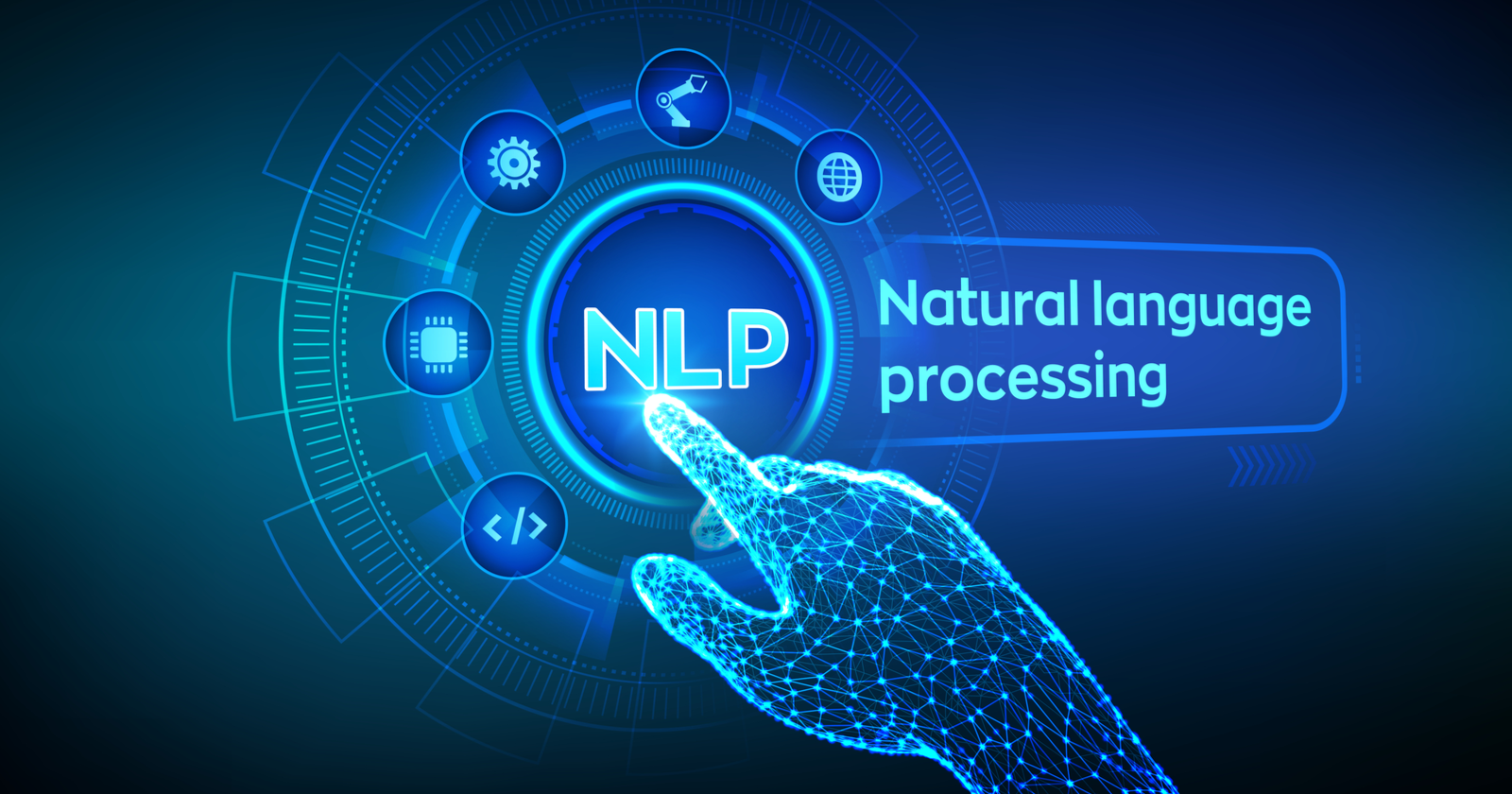# NLP In TensorFlow Using Transfer Learning.

Original article was published by Sailaja Karra on Deep Learning on Medium# NLP In TensorFlow Using Transfer Learning.

Today we are going to talk about deep learning using TensorFlow especially NLP.

Natural Language Processing is a very good task that can be easily accomplished by deep learning.

Today we are going to look at how to do NLP using Tensorflow for this we need to look at embedding layers.

Embedding layers are how a deep learning model can look at various words in multi-dimensions. Imagine if we can group of bunch words together either as good words or bad words, as in the case of movie reviews we can have something like funny and entertaining together versus not funny or boring on the opposite side.

It is easy to actually write a deep learning model from scratch a small model that we can put together to run NLP but what I want to show specifically in this blog today is how we can use transfer learning for NLP.

For this, we actually use the Stanford glove hundred embedded layer and convert that into an embed a matrix and use that matrix to do the classification.

To use the Stanford glove model the first layer would be the usual embedding layer but with weights set to the embedding matrix, we created and also setting the variable trainable to False.

To create the embedding matrix first we need to read the sentences, tokenize them using the standard tokenizer, and then figure out the number of vocabulary size. Then we create a dictionary of the words and then look up the word in the Stanford Glove model thus producing the matrix. It’s a lot easier in code.

## Embedding Matrix code ###

`embeddings_index = {}with open('/content/glove_6b_100d.txt') as f:   for line in f:     values = line.split()     word = values     coefs = np.asarray(values[1:], dtype='float32')     embeddings_index[word] = coefsembeddings_matrix = np.zeros((vocab_size+1, embedding_dim))for word, i in word_index.items():  embedding_vector = embeddings_index.get(word)  if embedding_vector is not None:    embeddings_matrix[i] = embedding_vector`

With the embedding matrix ready here is how we create the embedding layer with weights assigned to the embedding matrix. This one line is where all the transfer learning magic happens.

## Embedding layer with Embedding matrix code

`model = tf.keras.Sequential([       tf.keras.layers.Embedding(                       vocab_size+1,                       embedding_dim,                       input_length=max_length,                       weights=[embeddings_matrix],                        trainable=False),tf.keras.layers.Dropout(0.2),tf.keras.layers.Conv1D(64, 5, activation='relu'),tf.keras.layers.MaxPooling1D(pool_size=4),tf.keras.layers.LSTM(64),tf.keras.layers.Dense(1, activation='sigmoid')])`

The rest of the cord is pretty simple. It is the same exact copy from the basic tensor flow model.

With this our model is ready and we can run our model and see how it performs.

##Run the model ###

`model.compile(              loss='binary_crossentropy',              optimizer='adam',              metrics=['accuracy'])print(model.summary())history = model.fit(              training_padded,              training_labels,              epochs=num_epochs,              validation_data=(testing_padded, testing_labels),              verbose=2)`

As expected the model performs better with transfer learning and also as you can see the loss over time decreases and this helps with our NLP classification.